## Graphing Calculator 3D

Mods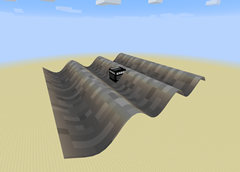Sine wave in Cartesian

A nice example of lighting/texturing.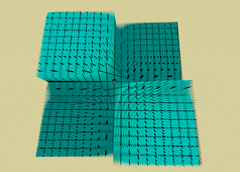Remainder function in Cartesian

Example of the complexity possible.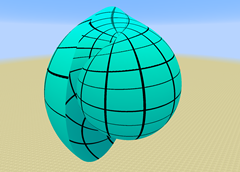r = phi, in Spherical

Nice texture stitching, mildly exotic coordinate system.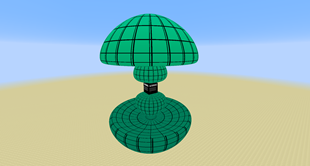Angle as a function of r, in Spherical

Any variable can be a function of any other, in any system.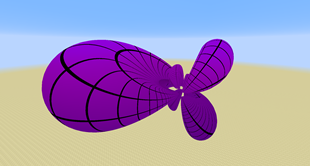Trig function in 6-Sphere

Unusual coordinate systems still look good.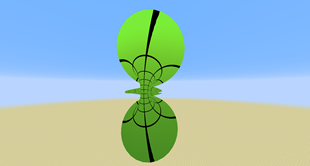tau = phi, in Toroidal

Very cool, very weird.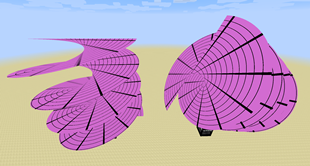h = theta, in Cylindrical

Copied with the Memory Card item, and rotated. Also scaled and translated.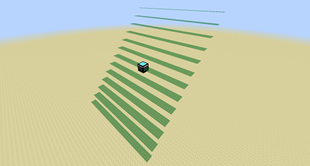y = int(x), in Cartesian

Good discontinuity detection, translucent graph.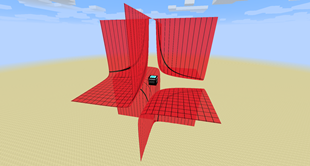y = 1 / (xz), in Cartesian

Asymptotes detected and disconnected.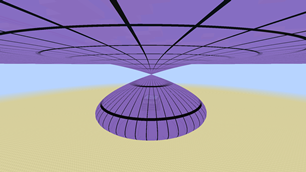nu = 1 / (sinh(mu)), in Oblate Spheroidal

Mostly just a cool graph.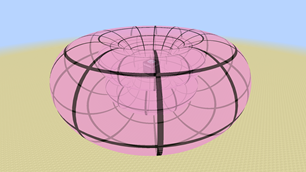tau = 1 / tanh(sigma), in Toroidal

Complicated graph, but well-textured.u = 1 / vw, in 6-Sphere

Helpful contour lines, scaled up for clarity. Another nice one.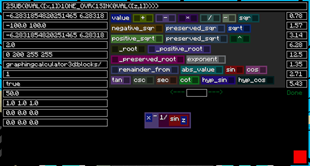The In-Game Gui

Lots of customization possible, and satisfyingly clicky equation blocks.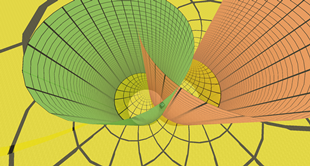Constants in Bipolar Cylindrical

Illustration of how you can use the mod to see and understand coordinate components.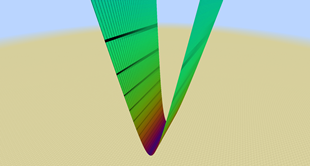Slope as color, in Cartesian

Illustration of graph-coloring by slope.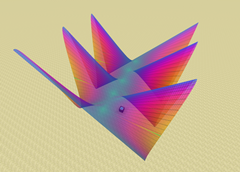Trig function with slope coloring, in Cartesian

Another example of slope coloring.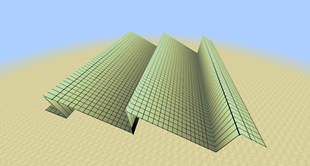Summation in Cartesian

A 100-term summation of sign waves, approximating a saw-wave.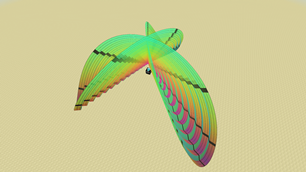Sigma summation in Parabolic

Another use of the series operations. (Also just too cool not to put here)